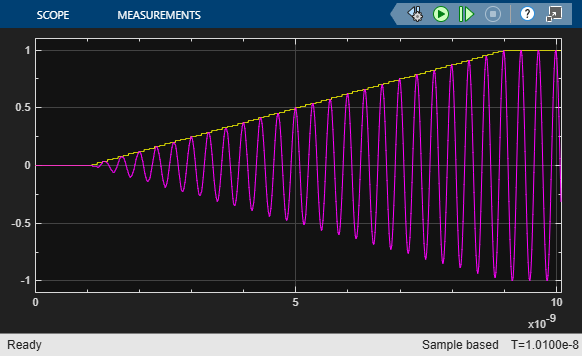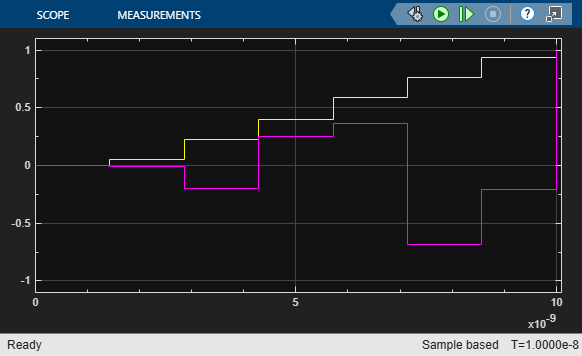Documentation

## Passband Signal Representation in Circuit Envelope

This model shows the relationship between two signal representations in RF Blockset™ Circuit Envelope: complex baseband (envelope) signal and passband (time domain) signal. The step size of a RF Blockset solver is usually much larger than the period of the carrier, so upsampling is necessary to construct a reasonable passband signal.

### System Architecture

The system consists of:

• Simulink blocks that generate a complex I+jQ input baseband signal.

• A RF Blockset Inport block that specifies the carrier frequency of the signal as f = 3GHz .

• A simple RF Blockset system that consists of an Amplifier with 0dB gain and matching 50 Ohm load (that is, its input and output signals are identical). It has two outports: baseband (where the complex envelope signal I+jQ is represented as magnitude and angle) and passband, where the actual time domain signal is reconstructed.

• A Scope block that displays baseband magnitude (that is, the envelope of the signal) versus the passband (actual) signal.

```model = 'simrfV2_passband'; open_system(model) ```### Definition of the Passband Signal

RF Blockset interprets the complex signal, as a modulation (envelope) of the sinusoidal carrier signal with a frequency. By default, RF Blockset assumes that the carrier signal is normalized (that is, its average power is equal to), so the passband signal is

``

With this definition, the average power of the signal is

``

In this example,is a ramp that goes fromtoand.

```scope = [model '/Scope']; set_param(scope, 'YMax','1.5'); set_param(scope, 'YMin','-1.5'); open_system(scope) sim(model); ```When the Normalize Carrier Power Option on the Configuration block is not selected, RF Blockset assumes thatrepresent the peak values of the carrier, that is

``

and the average power of the signal is therefore

``
```params = [model '/Configuration']; set_param(params, 'NormalizeCarrierPower', 'off') set_param(scope, 'YMax','1.1'); set_param(scope, 'YMin','-1.1'); sim(model); ```### Effects of the Normalize Carrier Power Option

It is very important to understand that when you change the Normalize Carrier Power option, RF Blockset changes the interpretation of the complex input/outputbaseband signal. Consider the simple case when the input baseband voltage is constant,and. The amplifier has a gain of 0dB, which means that the output signal is the same as the input.

When the Normalize option is checked, the output baseband voltage is equal to, the output passband voltage is, and the average power at the R = 50 Ohm load is.

When the Normalize option is unchecked, the output baseband signal does not change,, while the output passband signal is now, which means that the average power is.

In other words, for linear models the Normalize option does not affect the baseband output, but affects the actual passband signal and the average power formula.

Note that the zero carrier frequency is special: the passband and baseband representations forare always the same:### Simulation Step Size Versus Passband Output Step Size

In general, the RF Blockset simulation step is much larger than the period of the carrier, which allows faster simulation compared to regular methods. For such time steps the passband output is severely undersampled and exhibits aliasing effects. Set the Step Size value of the Configuration block to the large value 1e-8/7

```set_param(params, 'StepSize', '1e-8/7') sim(model); ```To obtain a realistic passband signal, resample the signal in the outport. Change the Step Size parameter of the Passband output block from -1 (which means that the step size is inherited from RF Blockset simulation) to 1e-11 .

```outport = [model '/Passband output']; set_param(outport, 'StepSize', '1e-11'); sim(model); ```Notes:

• Generating passband output at a higher rate (compared to RF Blockset simulation) requires resampling the signal's envelope. Current implementation uses a zero-hold resampling method that introduces "stepping" artifacts. Better interpolation techniques require delaying the output by several time steps.

• The 'auto' time step option is available on the RF Blockset Outport block (the time step is selected to resolve the highest output carrier frequency).

• Passband output might slow RF Blockset simulation because of the higher output sampling rate.

```bdclose(model) ```